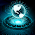## PHP CHALLENGE BY THAPA TECHNICAL

Q1:  Your challenge is to list all of the multiples of 12 which are less than or equal to 240.  You must complete this task twice, once using a While loop and once using a Do WHILE loop.
The multiples of 12 are 12,24,36,48,60,72,84,96,108,120,132,144,156,168,180,192,204,216,228 and 240.

Your answer format should be 12 * 1 = 12,  12 * 2 = 24, 12 * 3 = 36 etc till 12 * 20 = 240.

If you want some reference for it on how to do it. Feel free to watch my videos first on While loop, Do While Loop and For loop. Then I am 110% sure you can do it on your own. Here are the videos. You can directly watch it here only.

1.2.";
\$multiplier++;
}

?>

3.";
\$multiplier++;
}

?>

4.I'm Posting the right answer but it's not showing.

1.Sorry for that just take the pic or screen shot and paste it :)

5.My code is not visible properly in your comment section brother so here is my code "https://phpcodeanswer.blogspot.com/2020/02/answer-for-while-loop-num-12-multiplier.html"

6.// using while loop
";
\$a++;
}

?>
// using do while loop
";
\$a++;
}(\$a<=20);

?>

7.";
\$i++;
}while(\$i<=10);

// while(\$i<=10){
// echo \$i. "
";
// \$i++;
// }

?>

1.\$i = 11;

do{ echo \$i. "
";
\$i++;
}while(\$i<=10);

8.";
\$i++;
}

// 2 Task Do While loop Sol.
\$x=12;
\$i=1;
do {
echo \$x." * ".\$i." = ".\$x*\$i."";
\$i++;
}while(\$i<=20);

// 2 Task For loop Sol.
\$x=12;
for(\$i=1; \$i<=20; \$i++) {
echo \$x." * ".\$i." = ".\$x*\$i."";
}
?>

1.";
\$i++;
}

// 2 Task Do While loop Sol.
\$x=12;
\$i=1;
do {
echo \$x." * ".\$i." = ".\$x*\$i."";
\$i++;
}while(\$i<=20);

// 2 Task For loop Sol.
\$x=12;
for(\$i=1; \$i<=20; \$i++) {
echo \$x." * ".\$i." = ".\$x*\$i."";
}
?>

2.Proper Not Display

9.10.11.";
\$i++;
}while (\$i <= 20);
?>

12.//while loop

";
}
?>

//Do while loop

";
}While(\$i<=20)
?>

13.";
\$mult++;
}
\$number++;
}

//With Do...While Loop
do{
if(\$number%12==0){
echo "12*".\$mult."=".(\$number*1);
echo "
";
\$mult++;
}
\$number++;
}
while(\$number<=240)

//With For Loop
for(\$number=1;\$number<=240;\$number++){
if(\$number%12==0){
echo "12*".\$mult."=".(\$number*1);
echo "
";
\$mult++;
}
}

?>

14.There is something wrong with comment section- It's not proper displaying!

15.Nice vedio sir

16.";
\$i+=12;
\$j++;
}

?>

17.18.";
\$i +=\$k;

}

?>

19.20.21.";
\$x++;
}while(\$y*\$x <= 240)
?>